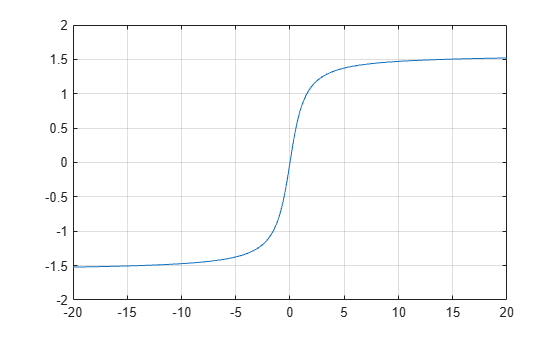# atan

## Syntax

``Y = atan(X)``

## Description

example

````Y = atan(X)` returns the Inverse Tangent (tan-1) of the elements of `X` in radians. The function accepts both real and complex inputs. For real values of `X`, `atan(X)` returns values in the interval [-π/2, π/2].For complex values of `X`, `atan(X)` returns complex values. ```

## Examples

collapse all

Find the inverse tangent of a value.

`atan(0.8)`
```ans = 0.6747 ```

Find the inverse tangent of the elements of vector `x`. The `atan` function acts on `x` element-wise.

```x = [0.5i 1+3i -2.2+i]; Y = atan(x)```
```Y = 1×3 complex 0.0000 + 0.5493i 1.4615 + 0.3059i -1.2019 + 0.1506i ```

Plot the inverse tangent function over the interval $-20\le x\le 20$.

```x = -20:0.01:20; plot(x,atan(x)) grid on```## Input Arguments

collapse all

Tangent of angle, specified as a scalar, vector, matrix, or multidimensional array. The `atan` operation is element-wise when `X` is nonscalar.

Data Types: `single` | `double`
Complex Number Support: Yes

collapse all

### Inverse Tangent

The inverse tangent is defined as

`${\mathrm{tan}}^{-1}\left(z\right)=\frac{i}{2}\mathrm{log}\left(\frac{i+z}{i-z}\right).$`

## Version History

Introduced before R2006a